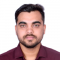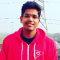## Remove or adapt the border of the frame of legend using matplotlibUpdated on 15-Mar-2021 07:41:58

801 Views

To remove or adapt the border of the frame of legend we can follow the following steps −Set the X-axis label using the plt.xlabel() method.Set the Y-axis label using the plt.ylabel() method.Plot the lines using the plt.plot() method with [9, 5], [2, 5] and [4, 7, 8] array.Initializing two variables; location = 0 for the best location and border_drawn_flag = True (True, if border to be drawn for legend. False, if border is not drawn).Use the plt.legend() method for the legend and set the location and border_drawn_flag accordingly to get the perfect legend in the diagram.plt.show() method would help to ... Read More

## How to plot a histogram using Matplotlib in Python with a list of data?Updated on 15-Mar-2021 07:40:11

11K+ Views

To plot a histogram using Matplotlib, we can follow the steps given below −Make a list of numbers and assign it to a variable x.Use the plt.hist() method to plot a histogram.Compute and draw the histogram of *x*.We can pass n-Dimensional arrays in the hist argument also.To show the plotted figure, use the plt.show() method.Examplefrom matplotlib import pyplot as plt x = [300, 400, 500, 2000, 10] plt.hist(x, 10) plt.show()Output

## How to display multiple images in one figure correctly in matplotlib?Updated on 15-Mar-2021 07:38:35

3K+ Views

To display multiple images in one figure, we can follow the steps given below −Initialize the number of rows and cols. nrows*ncols subplot will be created in the current figure. nrows = 2 and ncols = 2, i.e., 2*2 = 4 subplots can be created.Now add the figures at different indices from 1 to 4.Use plt.subplot(2, 2, 1) to add new images, i.e., pie at index 1.To plot a pie chart, pass a list of numbers. Pie charts will be split into the size of list and %age section will depend upon the values in the list.Set the title of ... Read More

## How to pick a new color for each plotted line within a figure in matplotlib?Updated on 15-Mar-2021 07:37:02

682 Views

To pick a new color for each plotted line within a figure, use the following steps −Setup X-axis and Y-axis labels for the diagram.Set the current .rc parameters. For axes facecolor, the group is axes.Use a cycler to set the color for the group of lines. The color list consists of ‘r’ for red, ‘g’ for green, ‘b’ for blue, and ‘y’ for yellow.Cycler class helps to create a new Cycler object from a single positional argument, a pair of positional arguments, or the combination of keyword arguments.Plot the number of lines with different colors.Use plt.show() to show the figure.Exampleimport ... Read More

## Hide axis values but keep axis tick labels in matplotlibUpdated on 15-Mar-2021 07:34:08

6K+ Views

To hide the axis value but to keep the axis tick labels, we can perform the following steps −Plot a line using the plot( ) method.Set X and Y labels using x label and y label methods.Using plt.gca(), get the current axis, creating one if necessary.Use xaxis.set_ticklabels() with an empty list.Use yaxis.set_ticklabels() with an empty list.To show the diagram, use the plt.show() method.Exampleimport matplotlib.pyplot as plt plt.plot([0, 5], [0, 5]) plt.ylabel("Y-axis ") plt.xlabel("X-axis ") ax = plt.gca() ax.axes.xaxis.set_ticklabels([]) ax.axes.yaxis.set_ticklabels([]) plt.show()OutputRead More

## Saving images in Python at a very high qualityUpdated on 15-Mar-2021 07:32:19

4K+ Views

To save the images in Python with very high quality, you need to follow the steps given below −Create fig and ax variables using subplots method, where default nrows and ncols are 1.Plot the lines using plot() method.We can add axes labels using ylabel() and xlabel().To get a high-quality image, we can use .eps image format.You can increase the dot per inch value, i.e., dpi.Using savefig() method, we can save the image locally.To show the figure, use plt.show().Exampleimport matplotlib.pyplot as plt fig, ax = plt.subplots() plt.plot([0, 5], [0, 5]) plt.ylabel("Y-axis ") plt.xlabel("X-axis ") image_format = 'eps' ... Read More

## How to move the Y-axis ticks from the left side of the plot to the right side in matplotlib?Updated on 15-Mar-2021 07:28:01

4K+ Views

To shift the Y-axis ticks from left to right, we can perform the following steps −Create a figure using the figure() method.Using the above figure method, create the axis of the plot, using add_subplot(xyz), where x is row, y is column, and z is index.To shift the Y-axis ticks from left to right, use ax.yaxis.tick_right() where ax is axis created using add_subplot(xyz) method.Now plot the line using plot() method, with given x and y points, where x and y points can be created using np.array() method.Set up x and y labels, e.g., X-axis and Y-axis , using xlabel and ylabel ... Read More

## Plotting regression and residual plot in MatplotlibUpdated on 23-Feb-2021 18:14:13

1K+ Views

To establish a simple relationship between the observations of a given joint distribution of a variable, we can create the plot for the regression model using Seaborn.To fit the dataset using the regression model, we have to first import the necessary libraries in Python.We will create plots for each regression model, (a) Linear Regression, (b) Polynomial Regression, and (c) Logistic Regression.In this example, we will use the wine quality dataset which can be accessed from here, https://archive.ics.uci.edu/ml/datasets/wine+qualityExampleimport matplotlib.pyplot as plt import seaborn as sns from scipy.stats import pearsonr sns.set(style="dark", color_codes=True) #import the dataset wine_quality = pd.read_csv('winequality-red.csv', delimiter=';') #Plotting ... Read More

## How to manage image resolution of a graph in MatplotlibUpdated on 23-Feb-2021 06:25:45

920 Views

An Image contains a 2-D matrix RGB data points which can be defined by the dots point per inch [ DPI ] of the image. The resolution of the image is important because a hi-resolution image will have much more clarity.We have a method ‘plt.savefig()’ in Matplotlib which determines the size of the image in terms of its pixels. Ideally it is having an ‘dpi’ parameter.Let’s see how we can manage the resolution of a graph in Matplotlib.Exampleimport matplotlib.pyplot as plt import numpy as np #Prepare the data for histogram np.random.seed(1961) nd = np.random.normal(13, 5, 1000) #Define the ... Read More

## How to provide shadow effect in a Plot using path_effect attribute in MatplotlibUpdated on 23-Feb-2021 06:25:32

697 Views

In order to provide path effects like shadow effect in a plot or a graph, we can use the path_effect attribute.For example, let’s see how we can use the path_effect attribute in Matplotlib add a shadow effect to a sigmoid function.import matplotlib.pyplot as plt import numpy as np from matplotlib.patheffects import PathPatchEffect, SimpleLineShadow, NormalNow let us define the size of the figure and plot the sigmoid function, plt.style.use('seaborn-deep') plt.subplots(figsize=(10, 10))Let us define the datapoints for the plot, x = np.linspace(-10, 10, 50) y = 1+ np.exp(-x))Let us define the shadow property in the plot, plt.plot(x, y, linewidth=8, color='blue', path_effects= [SimpleLineShadow(), ... Read More